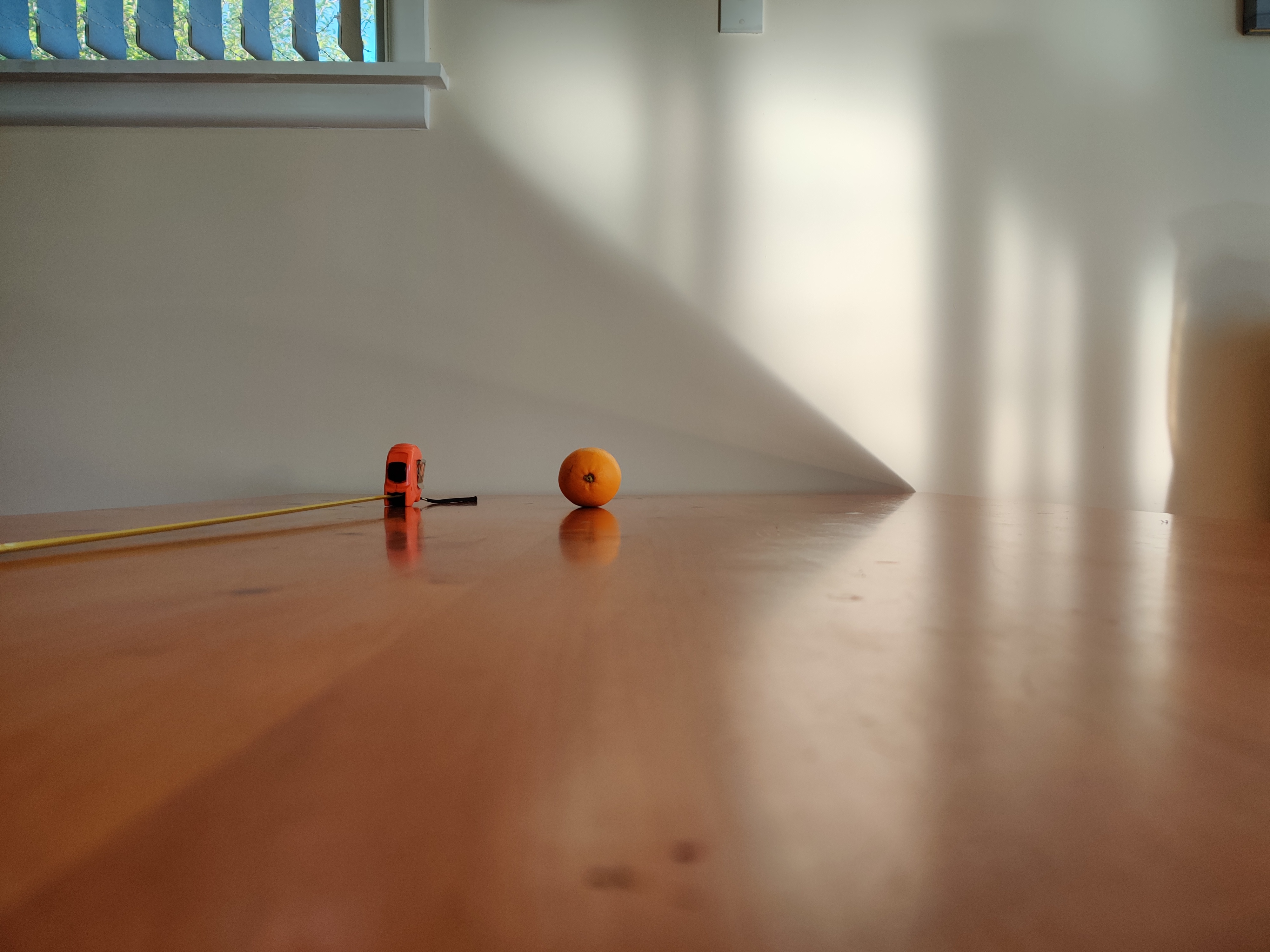Getting Distance From Stereo Images

Instructions (hide/show)

Take two side-by-side pictures of an object. Make sure the direction of the pictures are parallel, and that the object is between the two camera positions. Note down the precise distance between the camera positions and the distance between the camera plane and the object plane. Upload the two pictures and click on the common feature of the object in both pictures. Enter your camera's angle-of-view and the distance between the two camera positions, and click 'Calculate distance'. Note that resizing the window will reset the points as this can affect the size of the pictures. The values are inputted into the equation below.

The orange in the supplied images was 1 metre away. How close is the calculation?

$${D =\frac{Bx_0}{2tan(\frac{\theta_0}{2})(x_1-x_2)}}$$

Left CameraRight CameraLeft Width (x0): px

Left X (x1):

Left Y:

Right Width (x0): px

Right X (x2):

Right Y:

Angle of view (θ0)
Degrees
Distance between pictures (B)

Calculated distance: